Latest Banking jobs   »

# Reasoning Ability Quiz For RBI Grade B/ ECGC PO/ SIDBI Grade A Prelims 2022- 21st May

Directions (1-5): Study the following information carefully and answer the given questions.
J, K, L, M, N, O, P and Q are eight friends sit around a circular table for a meeting. Each of them used different types of vehicles to reach the meeting viz. Cycle, Truck, Car, Train, Scooter, Bus, Ship and Airplane but not necessarily in the same order. Four of them face towards the center and four of them are facing away from the center. Both the immediate neighbors of N face away from the center and one of them uses a Car and one of them uses a Train. N sits third to the right of O who used Scooter. L sits third to the left of O. The one who used Car sits opposite to O. The one who used Truck is not the immediate neighbor of O and faces away from the center. J sits second to the left of L. J neither used Ship nor an Airplane to reach the meeting. The one who used Ship is an immediate neighbor of Q and O. K who did not use truck faces away from the center. N faces towards the center and used Cycle. M faces away from the center. Both the immediate neighbors of M face towards the center.

Q1. Who among the following used Bus?
(a) Q
(b) J
(c) L
(d) M
(e) None of these

Q2. Who among the following sits fourth to the right of the one who used truck?
(a) Q
(b) N
(c) P
(d) O
(e) None of these

Q3. Who among the following sits third to the right of L?
(a) J
(b) K
(c) L
(d) Q
(e) O

Q4. Which of the following combinations is true?
(a) J – Scooter
(b) K – Train
(c) P – Ship
(d) O – Car
(e) None of these

Q5. Who among the following is an immediate neighbor of both P and J?
(a) Q
(b) N
(c) M
(d) O
(e) None of these

Q6. Which of the following symbols should replace the question mark (?) in the given expression in order to make the expressions A > B definitely true and E > B definitely false?
A ≥ C ? B < D ? E
(a) ≥, ≤
(b) >, =
(c) <, >
(d) ≥ , >
(e) none of these

Q7. Which of the following should be placed in the blank spaces respectively (in the same order from left to right) in order to complete the given expression in such a manner that makes the expression A > E as well as E ≤ R definitely true?
H _ A _ R _ S _ E
(a) =, =, ≥, ≥
(b) >, ≥, =, >
(c) >, <, =, ≤
(d) >, =, >, ≥
(e) >, >, ≥, =

Q8. Which of the following symbols should be placed in the blank spaces respectively (in the same order from left to right) in order to complete the given expression in such a manner that makes the expression H > S and A > E definitely false?
H _ A _ R _ S _ E
(a) <, <, <, =
(b) <, =, =, >
(c) >, =, =, <
(d) >, =, =, ≥
(e) >, >, =, <

Directions (9-10): In these questions, relationship between different elements is shown in the statements. The statements are followed by conclusions. Study the conclusions based on the given statements and select the appropriate answer. Give answer-

Q9.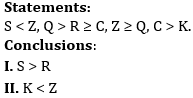(a) If only conclusion I is true
(b) If only conclusion II is true
(c) If both conclusions I and II are true.
(d) If either conclusion I or II is true
(e) If neither conclusion I nor II is true.

Q10.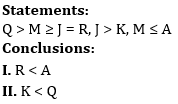(a) If only conclusion I is true
(b) If only conclusion II is true
(c) If both conclusions I and II are true.
(d) If either conclusion I or II is true
(e) If neither conclusion I nor II is true.

Directions (11-13): Study the following information carefully and answer the given questions.
There are seven members in a family i.e. A, B, C, D, E, F and G. Among them only two are married couple and only three are males. A who is unmarried and F are siblings. B’s husband has two children. D is father of F. G is niece of A who is not a male member. B is mother of C who is unmarried. E is not married to F or G.

Q11. Who among the following is Aunt of C?
(a) D
(b) E
(c) G
(d) A
(e) None of these

Q12. Who among the following is grandmother of G?
(a) E
(b) F
(c) B
(d) C
(e) None of these

Q13. How B related to A?
(a) Sister
(b) Aunt
(c) Mother
(d) Sister-in-law
(e) Brother

Directions (14-15): Read the following information carefully and answer the questions given below.
A # B means ‘A is son of B’
A \$ B means ‘A is wife of B’
A * B means ‘A is sister of B’
A @ B means ‘A is brother of B’
A & B means ‘A is father of B’

Q14. If A # B \$ C @ D & E, which of the following is true?
(a) E is the sister of C
(b) C is an aunt of A
(c) D is the brother-in-law of B
(d) A is the son of D
(e) None is true

Q15. Which of the following shows that R is the daughter-in-law of S?
(a) Q & R * T @ P # U * S
(b) Q \$ R & T @ P * S & U
(c) R & Q * T @ P \$ U & S
(d) Q & R \$ T @ P # S & U
(e) None of these

Solutions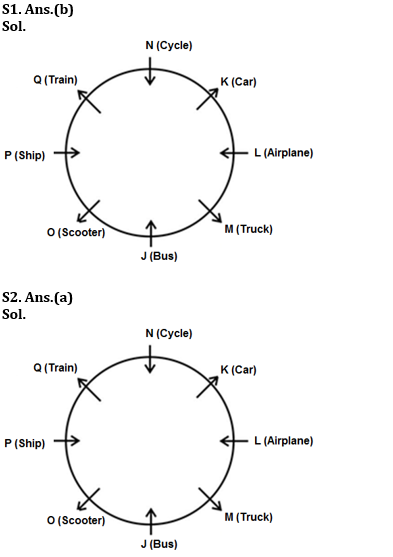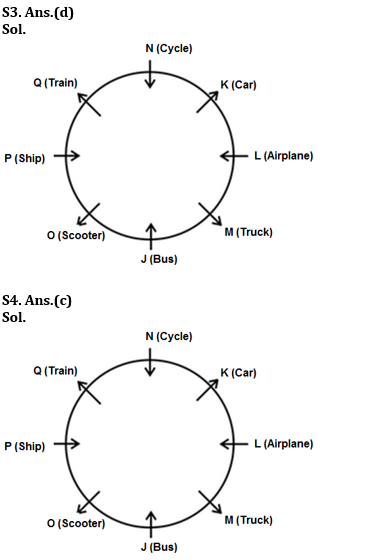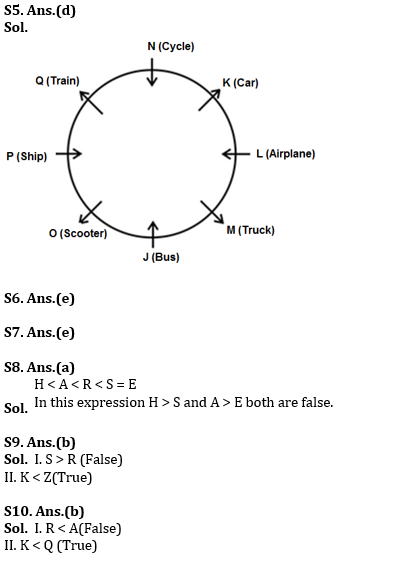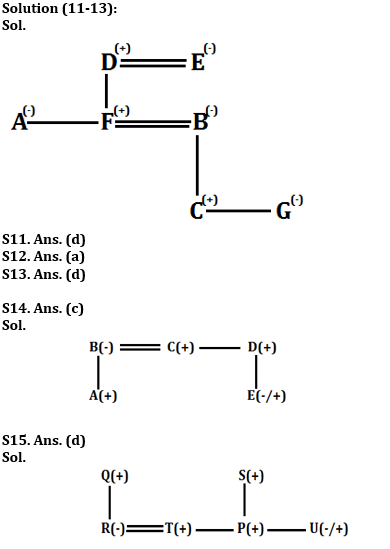#### Congratulations!